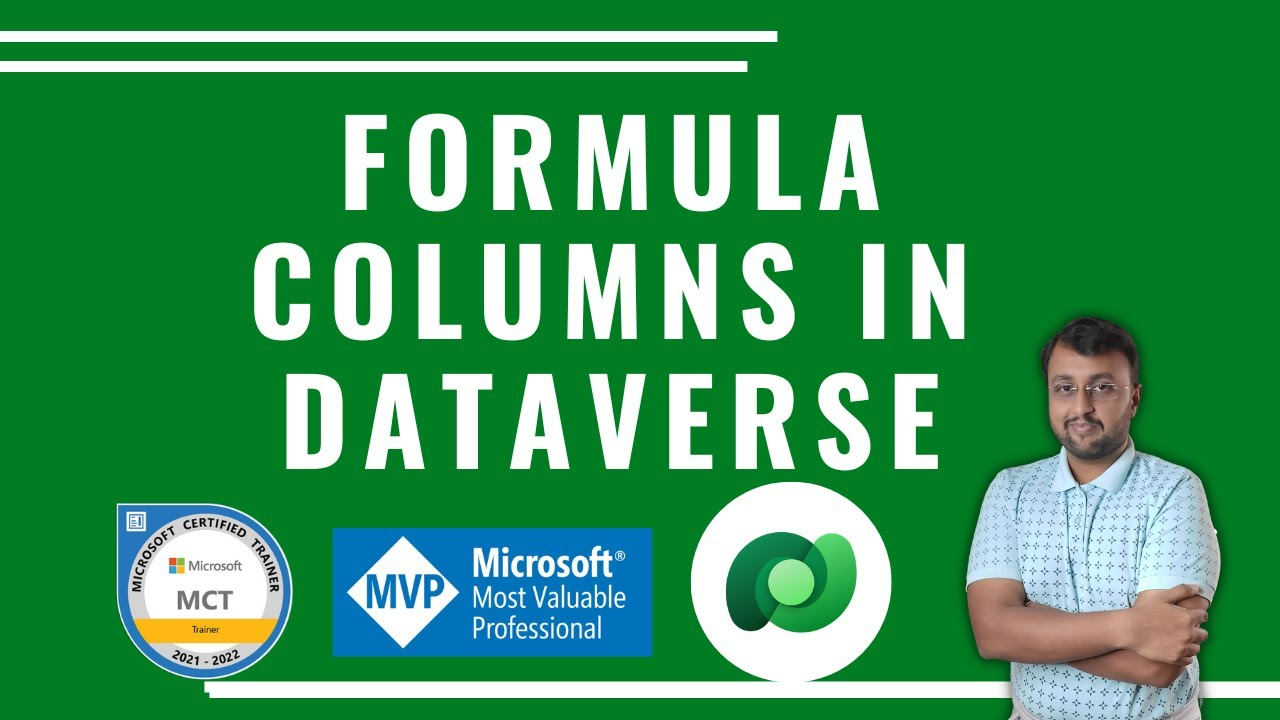en | deDec 14, 2022 7:00 AM

# Formula Column in Dataverse

## During this short video, we will learn about Formula Columns in Dataverse.

During this short video, we will learn about Formula Columns in Dataverse. Formula columns are columns that display a calculated value in a Microsoft Dataverse table.

Formulas use Power Fx, a powerful but human-friendly programming language. Build a formula in a Dataverse formula column the same way you would build a formula in Microsoft Excel. As you type, Intellisense suggests functions and syntax, and even helps you fix errors.

## Key Takeaways:

1. Create Formula Columns in Dataverse
2. Add Power Fx in Dataverse Column
3. Work with formula columns
4. Use PowerFX in Formula Columns for Dataverse

## Chapters:

00:00 Start

00:30 Formula Column in Dataverse

02:35 Test Values

03:10 Subscriber!

HubSite 365 Apps